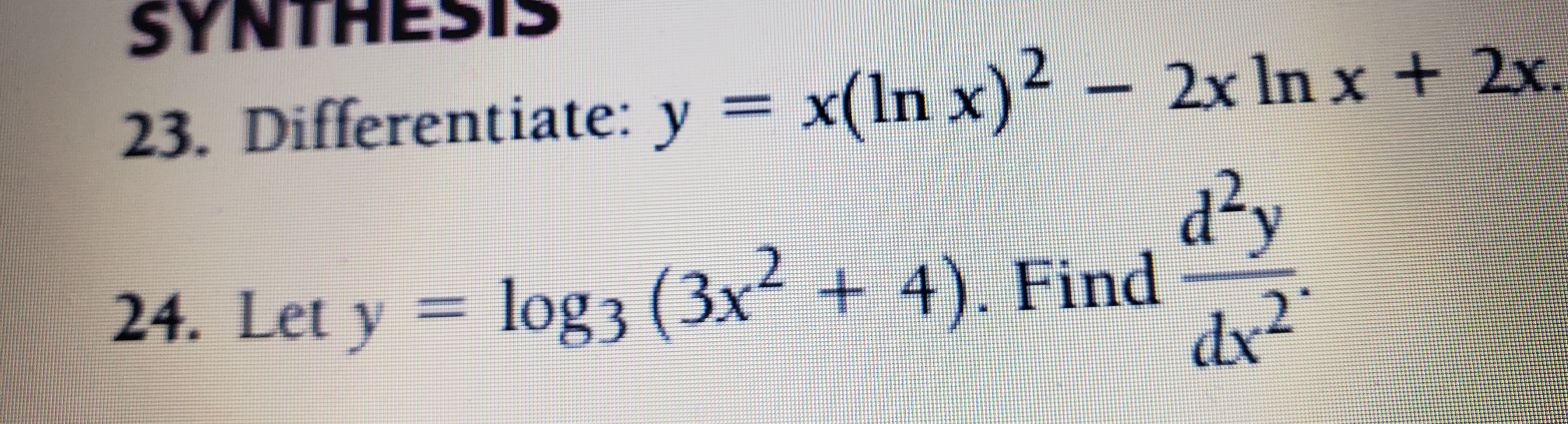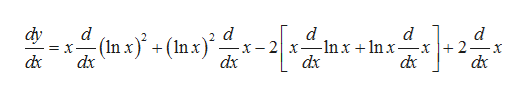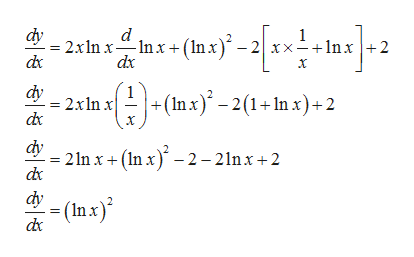# SYNTHESIS23. Differentiate: y = x(ln x)²– 2x In x + 2x.%3Dd²ylog 3 (3x² + 4). Finddx24. Let y =2.

Question
25 viewshelp_outlineImage TranscriptioncloseSYNTHESIS 23. Differentiate: y = x(ln x)² – 2x In x + 2x. %3D d²y log 3 (3x² + 4). Find dx 24. Let y = 2. fullscreen
check_circle

Step 1

Given function is

Step 2

We will use product rule of differentiation to find dy/dx which states that  if f(x)=u(x)v(x), then f'=uv'+u'vhelp_outlineImage Transcriptionclosex (nx}* + (Inx)* dy d X ]+2- dx - (In x}. -In x + In x- dx dx dx dx dx fullscreen
Step 3

Thus, we h...help_outlineImage Transcriptionclosedy 2xln xInx + (In.x) dx -2 xx+1n x +2 dx dy cla a-(mx)' - 2(1+ In x) +2 +(Inx)° – 2(1+ln x) + 2 = 2xln dx dy 2 In x + (In x) - 2– 21nx + 2 dx dy (In x)² de fullscreen

### Want to see the full answer?

See Solution

#### Want to see this answer and more?

Solutions are written by subject experts who are available 24/7. Questions are typically answered within 1 hour.*

See Solution
*Response times may vary by subject and question.
Tagged in

### Derivative